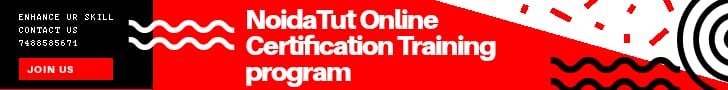FREE E LEARNING PLATFORM

# Check Prime Number using FunctionA number which is only divisible by itself and 1 is known as prime number. For example 13 is a prime number because it is only divisible by itself and 1, while 14 is not a prime number because it is divisible by 2 and 7 along with the number itself and 1.

This program takes the input number and checks whether the number is prime number or not using a function.

### Checking prime number using function

In this program, we have created a function called isPrime(int) which takes integer number as input and returns a boolean value true or false.

The program takes the value of num (entered by user) and passes this value while calling isPrime() function. This function checks whether the passed value is prime or not, if the value is prime then it returns true, else it returns false.

```#include <iostream>
using namespace std;
bool isPrime(int num);
int main(){
int num;
bool flag;
cout<<"Enter any number(should be positive integer): ";
cin>>num;     flag = isPrime(num);
if (flag==true)        cout<<num<<" is a prime number";
else
cout<<num<<" is not a prime number";
return 0;  }
bool isPrime(int num){
bool flag=true;
for(int i = 2; i <= num / 2; i++) {
if(num % i == 0) {
flag = false;
break;
}      }
return flag;
}```

### Output

```Enter any number(should be positive integer): 9
9 is not a prime number```